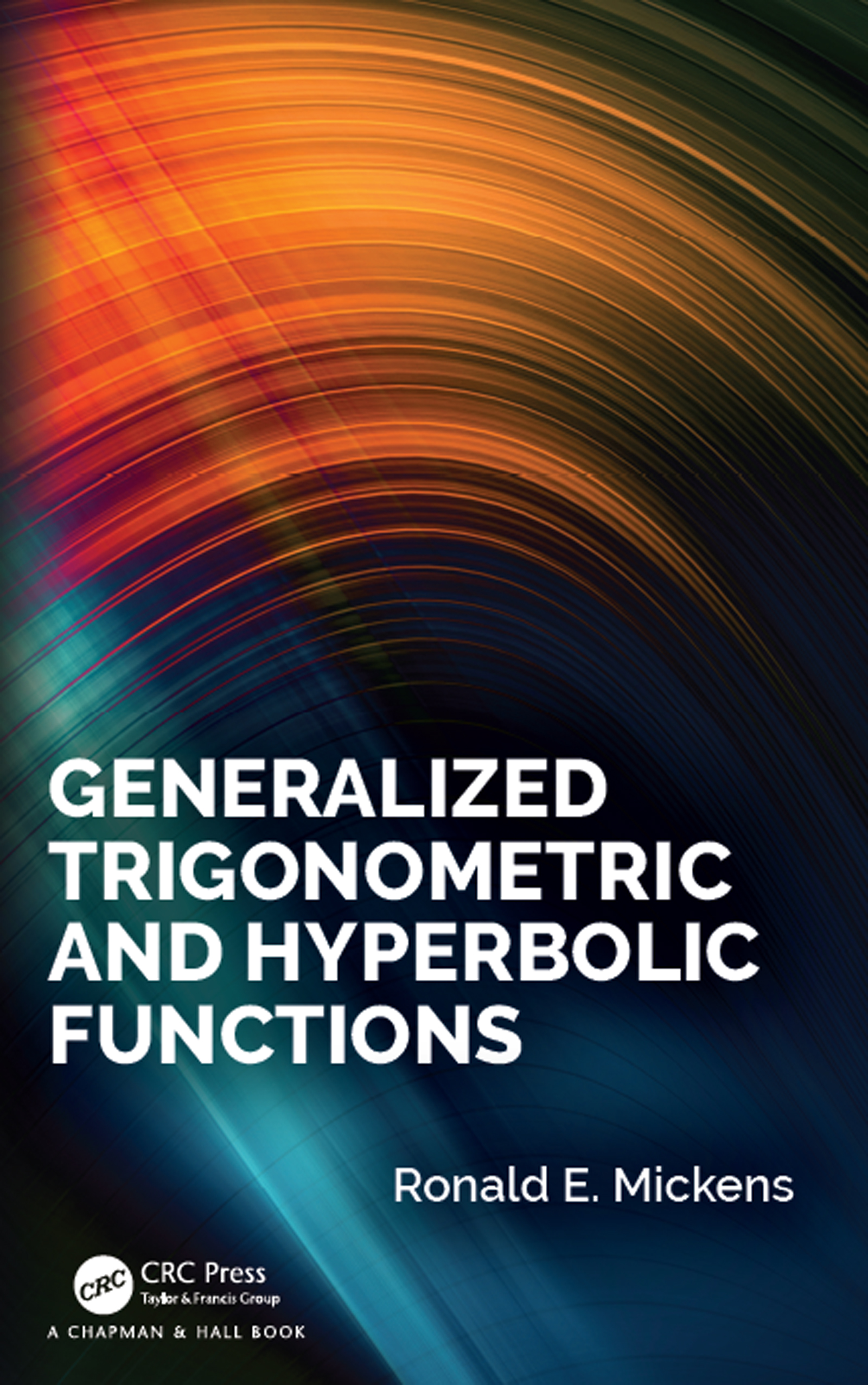# Generalized Trigonometric and Hyperbolic Functions

## 1st Edition

CRC Press

194 pages | 47 B/W Illus.

Hardback: 9781138333017
pub: 2019-01-14
SAVE ~\$29.99
\$149.95
\$119.96
x
eBook (VitalSource) : 9780429446238
pub: 2019-01-15
from \$28.98

FREE Standard Shipping!

### Description

Generalized Trigonometric and Hyperbolic Functions highlights, to those in the area of generalized trigonometric functions, an alternative path to the creation and analysis of these classes of functions. Previous efforts have started with integral representations for the inverse generalized sine functions, followed by the construction of the associated cosine functions, and from this, various properties of the generalized trigonometric functions are derived. However, the results contained in this book are based on the application of both geometrical phase space and dynamical systems methodologies.

Features

• Clear, direct construction of a new set of generalized trigonometric and hyperbolic functions
• Presentation of why x2+y2 = 1, and related expressions, may be interpreted in three distinct ways
• All the constructions, proofs, and derivations can be readily followed and understood by students, researchers, and professionals in the natural and mathematical sciences

1 TRIGONOMETRIC AND HYPERBOLIC SINE AND CO-SINE FUNCTIONS

2 ELLIPTIC FUNCTIONS

3 SQUARE FUNCTIONS

4 PARABOLIC TRIGONOMETRIC FUNCTIONS

5 GENERALIZED PERIODIC SOLUTIONS OF f(t)2+g(t)2 = 1

6 RESUME OF (SOME) PREVIOUS RESULTS ON GENERALIZED TRIGONOMETRIC FUNCTIONS 99

7 GENERALIZED TRIGONOMETRIC FUNCTIONS: |y|p +|x|q = 1

8 GENERALIZED TRIGONOMETRIC HYPERBOLIC FUNCTIONS: |y|p − |x|q = 1

10 FINALE